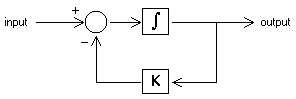﻿ 20-sim webhelp > Simulator > Running a Simulation > Copy States

# Copy States

To get the same model behavior, compared to the end of the previous run, we have to copy the states of a model to the initial values.

When you use the Copy States command of the Simulation menu, 20-sim overwrites the initial values of the functions ddt, int, limint and resint with their current output states. This can be done at the end of a simulation run or at any point where a simulation run was interrupted.

## Tip 1

You can reset the initial values to zero by using the Reset Initials command from the Simulation menu.

## Tip 2

For some models, behavior at some operating point or "steady state" is of interest. Use the Copy States command to create simulation that directly starts at working level:

 1 Run a simulation from t = 0 [s] until the operating point is reached. Use the Copy States command to store the states as new initial values. Now save the experiment using some special name.
 2 Now each time you open this experiment, simulations directly start at operating point.

States

Consider the following first order linear model:This model can be described by the dynamic equation:

output = int(0) + int( f(input,output) )

with:

int(0) = the initial value of the integral

f(input,output) = input - K*output

In more general terms this equation can also be described as:

state = int(0) + int(rate)

rate = f(input, state)

When we start to simulate this model at t = 0 [s] the value of the integral is zero. The state is therefore equal to the initial value int(0):

state(0) = int(0)

At the end of a simulation, the integral may be unequal to zero. The state is therefore equal to:

state(end) = int(0) + int(rate(end)).

In other words, the value of the state is characteristic for the behavior of the model. To start a new simulation with the same behavior, we have to change the initial value to:

int(0) = state(end)

This is valid for all dynamic models: The states of a model are characteristic for its behavior. To get the same behavior, compared to the end of a previous run, we have to copy the states to the initial values.